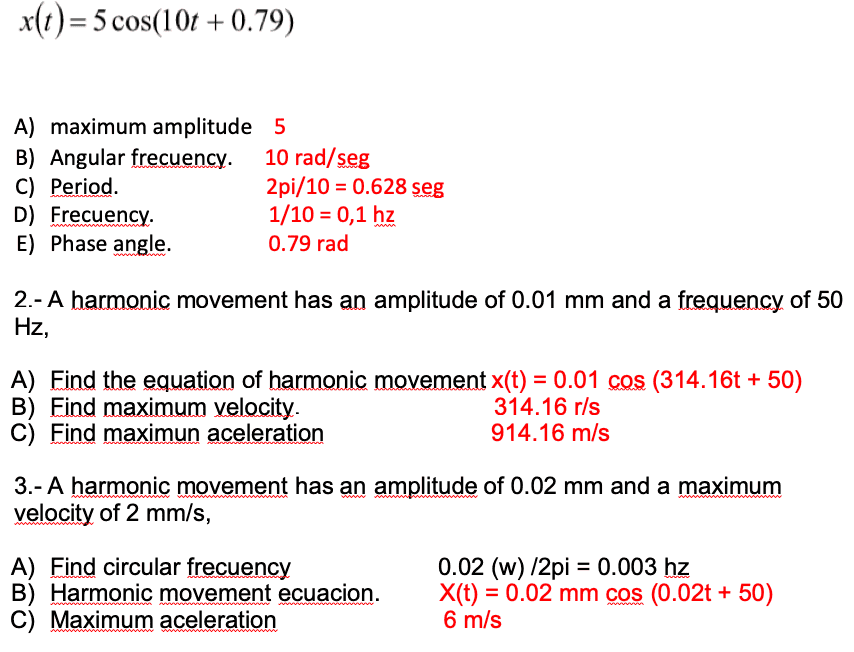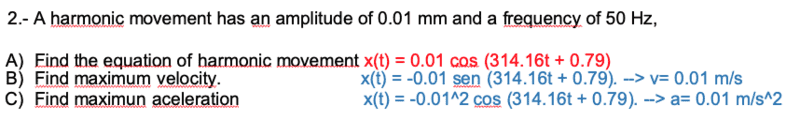# Simple harmonic motion (help please)

Homework Statement:
Determinate amplitude, velocity, aceleration, and ecuation of the principal ecuations (more information in the down image)
Relevant Equations:
1/T w/2piThe first ecuation values i am 99% that is correct. But, in the second and three problem i dont know if my results are ok. The problem number 2 i comprobate with the teacher that te aceleration its correct, so, with this i calculate the velocity.

I use like example the second problem for try resolve the 3rd problem, but causes me much problems.

#### Attachments

haruspex
Homework Helper
Gold Member
2020 Award
The first ecuation values i am 99% that is correct.
Except D.

The problem number 2 i comprobate with the teacher that te aceleration its correct, so, with this i calculate the velocity.
In a), why the +50?
In B), how did you calculate the velocity?
In C), despite your teacher's confirmation, I get a much smaller value.

I use like example the second problem for try resolve the 3rd problem, but causes me much problems.
I don't understand how you got any of those. Please explain your reasoning.

Except D.

In a), why the +50?
In B), how did you calculate the velocity?
In C), despite your teacher's confirmation, I get a much smaller value.

I don't understand how you got any of those. Please explain your reasoning.
a) Thats my mistake, i put hz in angle.
b) The velocity: the teacher just give us the ecuation 0.1cos (314.16t+angle), i the formula v= 2pi/w: 2pi/0.02
c) Maybe im wrong with the value copy, but i sure that say us 914.16 with m/s units.

For the 3rd problem i'm on my own with a single "class", i put the values in the ecuation of the best way i can.
For the circular frecuency i used the formula w/2pi.
For the aceleration i try to work the aceleration value with pi

b) have you had calculus? taking the derivative of the position x(t) will give you an equation for the velocity. The max amplitude should be apparent from that

c) m/s corresponds to a velocity, not acceleration. similar to (b) above, taking the derivative of v(t) will give you acceleration

b) have you had calculus? taking the derivative of the position x(t) will give you an equation for the velocity. The max amplitude should be apparent from that

c) m/s corresponds to a velocity, not acceleration. similar to (b) above, taking the derivative of v(t) will give you accelerationI tried make it better

#### Attachments

haruspex
Homework Helper
Gold Member
2020 Award
b) The velocity: the teacher just give us the ecuation 0.1cos (314.16t+angle), i the formula v= 2pi/w: 2pi/0.02
##100\pi## r/s is the angular velocity, which is constant. The question is asking for the maximum linear velocity, i.e. the maximum rate of change of x.
c) Maybe im wrong with the value copy, but i sure that say us 914.16 with m/s units.
The units are wrong for an acceleration, and if we correct the units to ##m/s^2## the number is wildly wrong. I want to know how you calculated it.
It sounds like someone gave you the answer but you wrote it down wrongly, and don't know how to find it for yourself. Is that right?
For the circular frecuency i used the formula w/2pi.
But where does the value of 0.02 r/s for w come from?
For the aceleration i try to work the aceleration value with pi

Here's how this stuff works:
##x(t)=A\sin(\omega t+\phi)##
Differentiate to find the velocity:
##v(t)=\frac{dx}{dt}=A\omega\cos(\omega t+\phi)##
Differentiate again to find the acceleration :
##a(t)=\frac{dv}{dt}=-A\omega^2\sin(\omega t+\phi)##

Do you understand those differentiation steps?